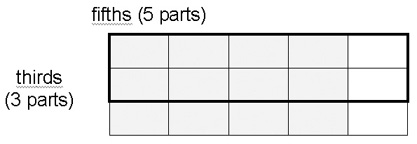# LAF Zone 4: Strategy Exploring

The information below describes the key characteristics and teaching implications for Zone 4 of the Learning and Assessment Framework for Multiplicative Thinking (LAF), including:

## How to use this resource

There are eight zones in the LAF. If your students are across several zones, you should access information for each of the zones where they are located.

Where there is a reference to:

## Key characteristics

Solves more familiar multiplication and division problems involving two-digit numbers. For example:

• Butterfly House parts c and d
• Packing Pots part c
• Speedy Snail part a.

Tends to rely on additive thinking, drawings and/or informal strategies to tackle problems involving larger numbers and/or decimals and less familiar situations. For example:

• Packing Pots part d
• Filling the Buses parts a and b
• Tables & Chairs parts g and h
• Butterfly House parts h and g
• Speedy Snail part c
• Computer Game part a
• Stained Glass Windows parts a and b.

Tends not to explain their thinking or indicate working.

Able to partition given number or quantity into equal parts and describe part formally (for example Pizza Party parts a and b). Can locate familiar fractions (for example, Missing Numbers part a).

Beginning to work with simple proportion. For example, can make a start, represent problem, but unable to complete successfully or justify their thinking (for example, How Far part a, School Fair parts a and b).

## Teaching implications – consolidation and establishment

Consolidate or establish the ideas and strategies introduced or developed in the previous zone

## Teaching implications – introduction and development

Multiplying and dividing larger whole numbers

Introduce more efficient strategies for multiplying and dividing larger whole numbers. These strategies work independently of models and are based on:

• doubling
• renaming the number of groups
• factors
• place-value

For example:

for dividing 564 by 8, think: 8 what’s are 560? 8 by 7 tens or 70, so 70 and 4 remainder

For example:

for 3908 divided by 10, rename as: 390 tens and 8 ones, so 390.8

Tenths as a new place-value part

Introduce by making or representing, naming and recording ones and tenths (see Booker et al, 2004 ).

Consolidate by:

• comparing
• ordering
• sequencing counting forwards and backwards in ones and/or tenths
• renaming.

Halving, thirding, fifthing strategies

Show students how to partition continuous quantities more generally using the halving, thirding, fifthing strategies.

For more information, see Siemon (2004) and Partitioning – The Missing Link in building Fraction Knowledge and Confidence (pdf - 199.51kb)

For example, recognise that:

• sixths can be made by halving and thirding (or vice versa)
• tenths can be made by fifthing and halving.

Use this knowledge to construct fraction diagrams (for example, region models) and representations (for example, number line) for common fractions and decimals including mixed numbers.

Renaming simple unlike fractions

Introduce informal, partition-based strategies for renaming simple unlike fractions. For example, recognise that thirds and fifths can be renamed by thirding and then fifthing (or vice versa) on a common diagram. An example of this type of diagram is shown below.Link to region model of multiplication (in this case 3 fives, or 3 parts by 5 parts) to recognise that thirds by fifths are fifteenths, so 2 thirds can be renamed as 10 fifths and 4 fifths can be renamed as 12 fifteenths.

Use strategies to informally add and subtract like and related fractions.

Key fraction generalisations

Use strategies that support the key fraction generalisations that recognise that:

• equal parts are necessary
• the total number of parts names the part
• as the total number of parts increases they get smaller.

This idea is crucial for the later development of more formal strategies for renaming fractions (see Zone 5) which relate the initial number of parts (3, thirds) to the final number of parts (15, fifteenths). In terms of factors, the number of parts has been increased by a factor of 5.

Metacognitive strategies

Introduce strategies to support:

• problem comprehension
• problem representation
• strategy monitoring and checking
• interpretation of outcomes relevant to context.

For more information, see the Siemon and Booker (1990) paper on Teaching and Learning For, About and Through Problem Solving (pdf - 462.19kb)

Simple proportion problems

Introduce techniques for dealing with these situations with simple proportion problems. For example:

• find for 1 then multiply or divide as appropriate
• using scale diagrams
• interpreting distances from maps.

## Learning plans

The following learning plans have been developed for this zone, to support targeted teaching after students have been assessed and located on the LAF

Zone 4 – Learning Plans Introducing (pdf - 63.28kb)